Examples

Chapter 15 Class 10 Probability
Serial order wise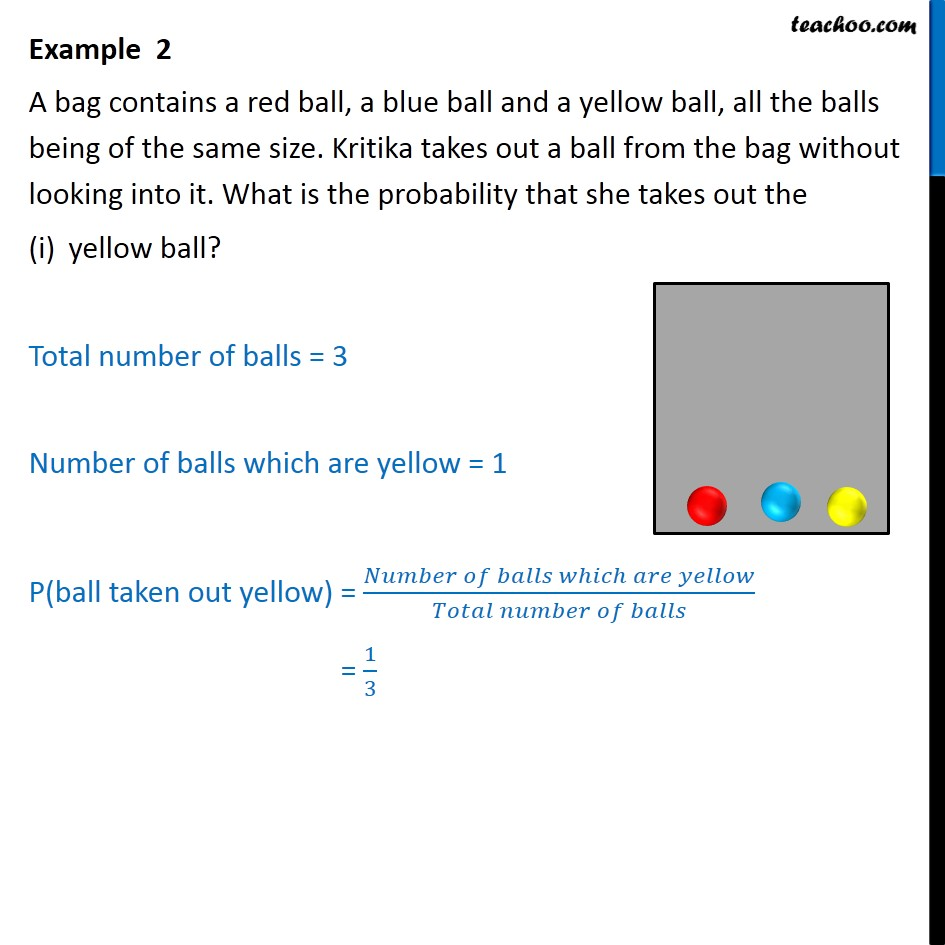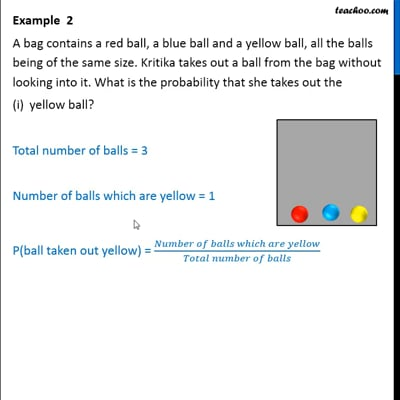This video is only available for Teachoo black users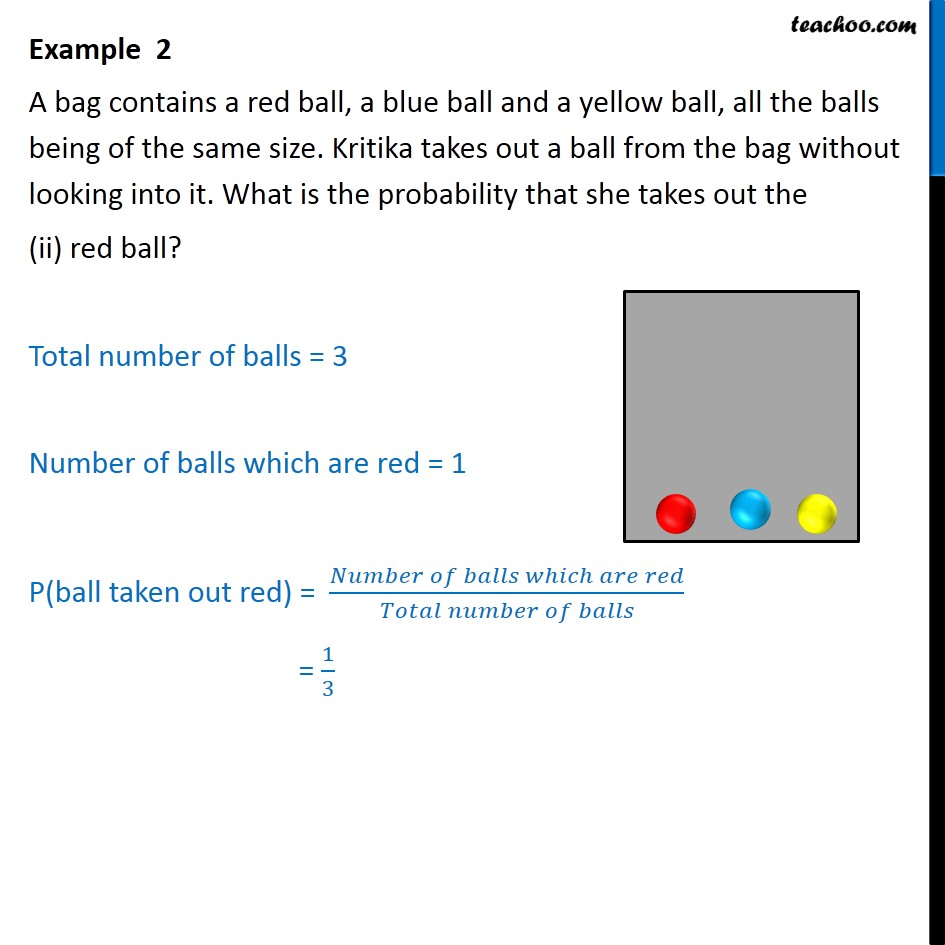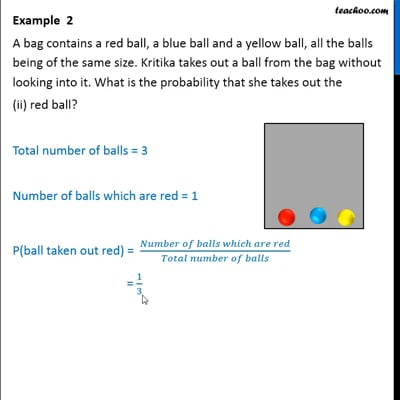This video is only available for Teachoo black users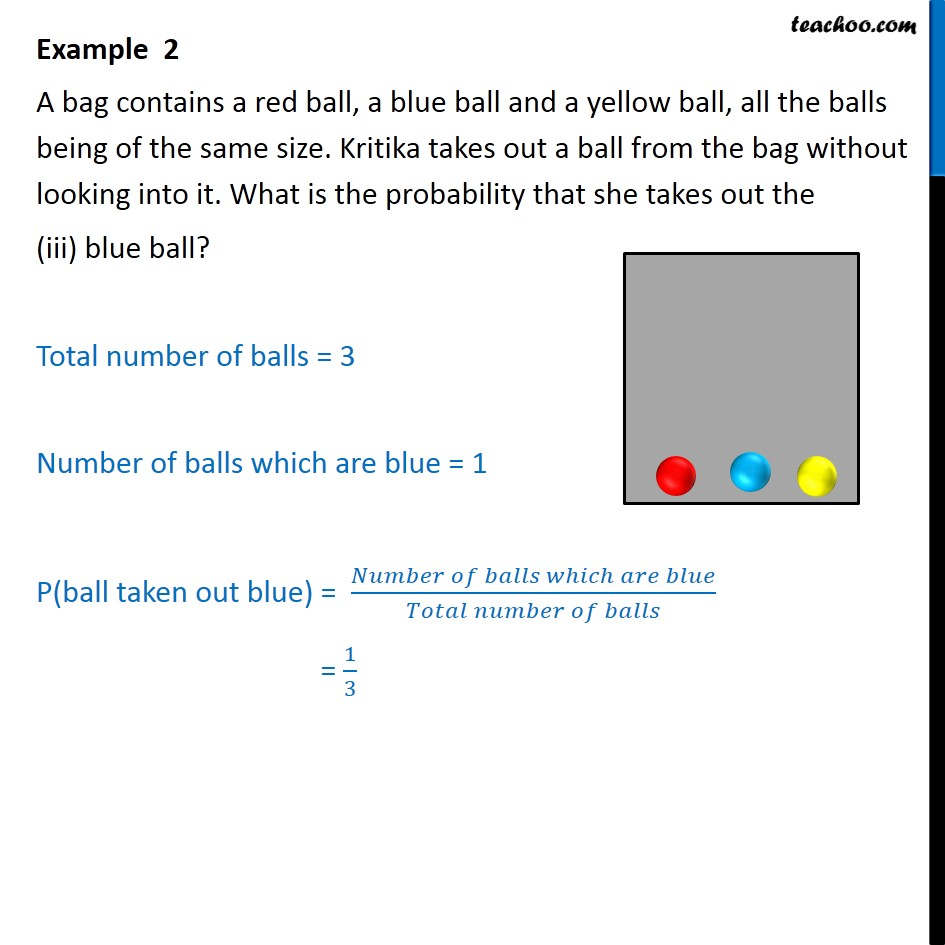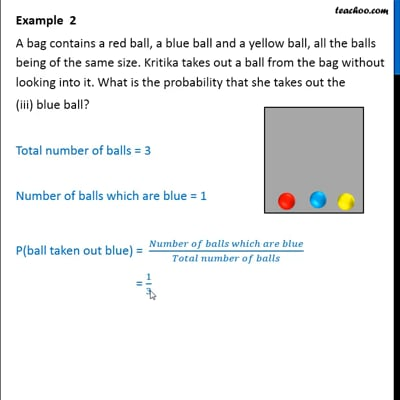This video is only available for Teachoo black users

Get live Maths 1-on-1 Classs - Class 6 to 12

### Transcript

Example 2 A bag contains a red ball, a blue ball and a yellow ball, all the balls being of the same size. Kritika takes out a ball from the bag without looking into it. What is the probability that she takes out the yellow ball? Total number of balls = 3 Number of balls which are yellow = 1 P(ball taken out yellow) = (𝑁𝑢𝑚𝑏𝑒𝑟 𝑜𝑓 𝑏𝑎𝑙𝑙𝑠 𝑤ℎ𝑖𝑐ℎ 𝑎𝑟𝑒 𝑦𝑒𝑙𝑙𝑜𝑤)/(𝑇𝑜𝑡𝑎𝑙 𝑛𝑢𝑚𝑏𝑒𝑟 𝑜𝑓 𝑏𝑎𝑙𝑙𝑠) = 1/3 Example 2 A bag contains a red ball, a blue ball and a yellow ball, all the balls being of the same size. Kritika takes out a ball from the bag without looking into it. What is the probability that she takes out the (ii) red ball? Total number of balls = 3 Number of balls which are red = 1 P(ball taken out red) = (𝑁𝑢𝑚𝑏𝑒𝑟 𝑜𝑓 𝑏𝑎𝑙𝑙𝑠 𝑤ℎ𝑖𝑐ℎ 𝑎𝑟𝑒 𝑟𝑒𝑑)/(𝑇𝑜𝑡𝑎𝑙 𝑛𝑢𝑚𝑏𝑒𝑟 𝑜𝑓 𝑏𝑎𝑙𝑙𝑠) = 1/3 Example 2 A bag contains a red ball, a blue ball and a yellow ball, all the balls being of the same size. Kritika takes out a ball from the bag without looking into it. What is the probability that she takes out the (iii) blue ball? Total number of balls = 3 Number of balls which are blue = 1 P(ball taken out blue) = (𝑁𝑢𝑚𝑏𝑒𝑟 𝑜𝑓 𝑏𝑎𝑙𝑙𝑠 𝑤ℎ𝑖𝑐ℎ 𝑎𝑟𝑒 𝑏𝑙𝑢𝑒)/(𝑇𝑜𝑡𝑎𝑙 𝑛𝑢𝑚𝑏𝑒𝑟 𝑜𝑓 𝑏𝑎𝑙𝑙𝑠) = 1/3